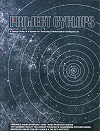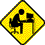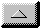Publications Department

Project Cyclops ErrataEven now, thirty years after the Project Cyclops design team was convened, readers are discovering minor errors and omissions in the Cyclops report. Larry Lesyna, who prepared the Errata sheet for Project Cyclops, Second Edition, has graciously volunteered to continue coordinating the compilation of corrections to this landmark manuscript. Readers of Project Cyclops are encouraged to email pertinent corrections to Larry at cyclops_errata @ setileague.org. Changes will be posted to this page once appropriately reviewed and verified.

Font Note: Proper display of the equations below requires use of a computer and browser with the Symbol font installed and enabled.

ERRATA (Project Cyclops, Second Edition)

page 1: In second column, second paragraph, replace "expanded" with "expended".

page 7: In Figure 2-2, the units of the x axis were omitted. The correct unit is 109 years.

page 11: In equations 4 and 5 unit "yr" should be included with 12 x 109.

page 13: In first column, fourth paragraph, replace "LaPlace" with "Laplace".

page 14: In caption under Figure 2-8, replace last sentence with "The bar in the upper right hand corner represents a photographic plate displacement corresponding to 0.01 arcseconds. The vertical axis is the star's motion as recorded on the photographic plate in units of microns. The size of the dots indicates the average error associated with any point. The solid curves represent the supposed path of the star derived from the observations."

page 15: In second column, third paragraph, replace "known to have dark companions" with "reported to have dark companions".

page 16: In Table 2-5 after "Mean Lifetimes of Atmospheric Components", add "(in years)". Replace "Oxygen" with "Neon".

page 17: In first column, second paragraph, replace "istopes" with "isotopes".

page 17: In second column, second paragraph, after "(r max / r min) = 1.5", add "where r is the distance from the planet to the Sun".

page 18: After equation (8) add "where r0 is the distance of the Earth from the Sun."

page 18: Above "The Origin of Life" replace "lower limit" with "lower mass limit".

page 25: In Figure 2-10, the heavy line for curve (4) should follow the x-axis until it reaches curve (2), then should follow curve (2) to reach a maximum at t = G + L. It then drops along the line to merge with the heavy dashed curve.

page 25: In second column, paragraph below Figure 2-10 replace "dotted curve" with "heavy dashed curve".

page 26: In second column, under definition of R* , add "T = age of our galaxy".

page 26: In equation (14) add "(in years)" after "L".

page 28: In Reference #3 replace "Shklovski" with "Shklovskii". In Reference #13 replace "Geophysic" with "Geophys."

page 33: In the first column, replace "Since the nearest star, a-Centauri, is 4 light-years away..." with "The nearest star, beyond the Sun, is the a-Centauri system. At four light-years away..." (Proxima Centauri is technically the closest extrasolar star).

page 37: In first paragraph under "Antenna Gain and Directivity", after "the same relation to the wavelength", add ", l".

page 37: In last paragraph, first column, replace "An antenna whose area is greater" with "An antenna whose effective area is greater".

page 38: In Figure 5-1 the units of the y-axis is "Kelvins".

page 40: In text above eq (18) replace "shot" with "shot noise".

page 41: In figure 5-2 replace x-axis number "0" with "10-1".

page 42: In equation 23, T is missing subscript "*".

page 43: In second column replace "Sychronous" with "Synchronous".

page 46: Below eq. 38 add "where Pn is the receiver noise".

page 46: In second column, above "Photon Detection" after "uncorrelated receiver noise" add a comma.

page 47: In paragraph below Figure 5-7 replace "Simlarly" with "Similarly".

page 47: In equation 47, numerator should be ln((t/T)pe).

page 47: In equation 48, subscript T is missing from n on left hand side. Delete "dn" from right hand side.

page 47: Below equation 49, "that is independent of n", bar belongs over the n.

page 49: In paragraph above "System B" replace "1360 degrees" with "1360 K". Replace "h" with "n".

page 50: In Table 5-3, replace "Effective radiated power" with "Peak effective radiated power".

page 51: In Figure 5-8 replace "2.4 x 10-4" with "2.4 x 10-3".

page 52: In first paragraph, after "Viterbi decoder", add ", where W is the energy per transmitted or signal bit".

page 53: In equation 1, replace second equality with an approximately equal sign.

page 53: Halfway down the right-hand column, 0.088 / pc3 should read 0.0188 / pc3.

page 54: In equation (3), the limits of integration (shown as - to + infinity) should be -R to +R.

page 54: In equation (3), the minus sign under the first radical should be a plus sign.

page 54: In equation (3), log [for common logarithm] should read ln [for natural logarithm].

page 55: In equation (5) and the prior paragraph, q refers to the half-angle of total beamwidth.

page 67: In second column, first paragraph, replace "10 sec" with "10 sec of arc".

page 71: In second column, second paragraph, replace "1-KHz" with "1-kHz".

page 75: In first column third paragraph, replace "500 kw" with "500 kW".

page 83: In first column below eq (10) add at end of definition for da, "in meters".

page 84: Under acknowledgements, replace "Lockeed" with "Lockheed".

page 88: In first column, third paragraph, after "(right circular)" add ", where j is the imaginary operator".

page 89: In second column, below eq (9) replace "Ta <= 7 degrees" with "Ta <= 7 K".

page 90: In the first column, replace " If n = 1 the amplitude has a paraboloidal distribution." with "If n = 2 the amplitude has a paraboloidal distribution."

page 95: In first column, second paragraph, replace first sentence with "As shown, the six up-converters associated with six bands in a single polarization are clustered in a 10 W capacity, 20 K closed cycle cryostat, while the other six up-converters corresponding to the orthogonal polarization are in a second cryostat."

page 101: In Figure 9-17 delete two horizontal arrows above the "Majority Logic" and " divide by 4" boxes.

page 112: In Figure 10-7, the horizontal axis should be labelled "Frequency, MHz".

page 116: In first column, third paragraph, replace "Rayliegh" with "Rayleigh".

page 126: Add "sec" after two equations for T, and add "Hz" after 5 x 105.

page 129: In paragraph below "Doppler Rate Compensation" add dot over n and add dot over 2Df.

page 133: In Figure 11-8 delete "0" over the second occurance of Z0.

page 133: Below eq (15) add "where Si(x) = integral from 0 to x of sin y dy/y".

page 135: In first column, in definition for gb, replace "cost per bit or data sample stored" with "cost per data sample stored".

page 135: In second column, in expression for gb, replace "\$2x10-4" with "\$3 x 10-4".

page 136: In eq (23) numerator after first equality should be "ga m - 3 gd". Numerator after second equality should be "ga Ta Df - 3 gd".

page 136: In section that begins "1. 1 Hz channels, N = 106", replace "104" with "106", replace "1640"with "16,700" and replace "40" with "408" (two occurences).

page 136: In second column, first sentence, replace "50 to 150 samples" with "100 to 500 samples".

page 137: In equation 24, insert "/" between (f - f0) and Df in numerator.

page 138: In second column, second paragraph, replace "preparing Figure 11-13" with "preparing Figure 11-14".

page 139: In first column, first paragraph, replace "Df = 0.1" with "Df = 0.1 Hz".

page 141: In first sentence replace "values of P" with "values of F".

page 141: Below Figure 11-15 replace "4 kHz" with "5 kHz".

page 143: In second column, above eq (47) replace "A(xi yi)" with "A(xi , yi)".

page 145: Above eq (56) extend radical sign over 4 n / p.

page 145: In second column, under 1. replace "Appendix D" with "Appendix M".

page 146: Above eq (58) extend radical sign over 4 n / p.

page 147: In first paragraph, extend radical sign over 4 n / p.

page 149: In eq (70) extend radical sign over 1 + r2/l2 and 1 + a2/l2.

page 151: In eq (71) the correct subscripts for G are "i,j".

page 151: In eq (72) in second factor including G, place comma between iu0 and jv0.

page 152: In Reference 5, add "1965," before "pp. 696-704".

page 153: In second column, first paragraph replace "bandwith" with "bandwidth".

page 161: In sentence above "The Four Search Phases" add "next to" before "last section of this chapter."

page 171: In first column, last sentence replace "the" with "The".

page 171: Under conclusion 14 "with greater a capability-to-cost ratio", delete "a".

page 173: In first column, replace last sentence with "The luminosity of the Sun (Lsun) is 3.9 x 1026 watts.

page 173: In second column, replace first sentence with "The bolometric magnitude, Mbol, of a star is a logarithmic measure of its luminosity."

page 173: In second column, in equation for Mbol, replace "3 x 1028" with "3 x 1028 watts".

page 173: In heading to Table A-1 replace "Te" with "Te", and "L/L0" with "L* / Lsun".

page 174: Under PLANETS, replace Pluto's period of rotation with "6.4h".

page 176: Replace "km/sec Mpc" with "km/ (sec Mpc)" in two instances.

page 183: In second column, above equation (C6) replace "we may rewrite equation (C4) as" with "we may rewrite equation (C3) as".

page 187: At end of second paragraph, add "The following results also assume that a single propagation mode is received."

page 187: In second column, after "A ei2p n0t", add "where A2 = Pr".

page 188: In second column, third paragraph, expression for qi bar should be a2 (h n/h) P bar.

page 188: In equation D7, second P0 in denominator should be squared.

page 189: In sentence above (D13), replace "expression (D5)" with "expression (D9)".

page 189: Below expression for H(f), the definition for G(f) is missing. It should be G(f) = 1 for |f|<W; G(f) = 0 for |f|>W.

page 189: In sentence above (D15) , replace "If an output filter" with "If no output filter".

page 191: In equation (D22), replace "hnW/h" with "hnB/h".

page 191: In paragraph above (D27), replace "As in the coherent case" with "As in the incoherent case".

page 193: In eq above (D37) replace "C(w" with "C(w)".

page 193: In last sentence, replace "variance" with "standard deviation".

page 194: Equation (D43) should read so = Po (m / n )1/2.

page 194: In equation below (D50), insert left parenthesis before first "ptf".

page 194: In first sentence, replace "variance" with "standard deviation".

page 194: Below (D43) replace "See eqs. (D18)" with "See eqs. (D23)".

page 194: In text below (D49) replace "s2" with "s02".

page 194: In eqn (D50) replace "P0 t" with "P0 / t".

page 195: In eqn (D53), after right hand side, add " = Pr2 / (hn / ht) Pr".

page 195: In paragraph below (D55), after "equation (D54)", insert ") with (D51),".

page 195: At the end of the paragraph following (D55) add "See equation 40 of Jakeman and Pike in J. Phys. A pp 115-125 (1969) for further details."

page 195: In last paragraph, replace "equations (D46) and (D47)" with "equations (D42) and (D43)".

page 195: Above (D58), replace "n bar / n bar*" with "n bar* / n bar".

page 195: In last line, second column, replace "Pr/Pn" with "P*/Pr".

page 196: In second column, first sentence replace "If m2 / ns b*" with "If (m2 / ns) b*".

page 196: In equation (D61), in numerator replace "m / ns" with "m2 / ns". Replace first occurence of "-4 m2 / n" with "+4 m2 / n". Replace "(1 + 2 m2/ns b*)" with "(1 - (m2/ns) b*". Replace "(1 + nr)" with "(nr - 1)". In demoninator, replace second occurence of "b*" with "b*2".

page 197: In equation (E9) add right parenthesis at end.

page 198: In equation (E12) replace "t" with "T".

page 201: In equations for Cmax and CAE, insert o after "70", "32", and "45".

page 203: In second column, second sentence, replace "cable area" with "cable area, A". In equation below, replace "(40 x 12)" with "40 ft". Replace "0.008 in." with "0.08 in."

page 205: In second column, second paragraph, replace "hydraulic jacks, that are properly arranged counterweights" with "hydraulic jacks, properly arranged with counterweights".

page 206: page number missing.

page 208: In first column, after "H-2b accomplishes this", add ", where j is the imaginary operator".

page 210: In second column, first sentence, replace "From equations (8) and (9)" with "From equations (I8) and (I9)".

page 214: In Figure K-1, last line, replace right arrow with minus sign. In Figure K-2 replace "NO" with "N0" (8 instances). In last line of Figure K-2, replace right arrow with minus sign.

page 217: In sentence above equation (L9) extend radical sign over "/p".

page 221: In first sentence, replace "noise signal" with "incoherent signal".

page 221: Below eqn (M1) replace "noise receiver A" with "noise from receiver A".

page 223: In equations (M23) and (M24) add "dB" to end of each equation.

page 224: Below equation (M27) add "where Si(x) = Integral from 0 to x of (sin y) dy/y".

page 224: Above equation (M28), add left parenthesis after "log" and right parenthesis at end. Add "dB" at end.

page 224: In equations above (M29) replace "d" with "l" (5 instances).

page 224: In equation (M31) replace "wi (t' - t)" with "wc (t' - t)".

page 225: Replace sentence starting with "Assume that" with "Assume that d = lc / 2d, that is, that we are attempting to image out to the half-power angle of each element, where d is the diameter of an individual antenna element".

page 226: Last sentence replace "Chapter 9" with "Chapter 11".

page 227: In definitions for d and h, replace "interests" with "intersects".

page 229: Below first equation add at end of sentence, "and j is the imaginary operator".

page 229: Second column, second equation, extend square brackets to include numerator and denominator.

page 229: In second part of expression for g(x,y) extend square brackets to include numerator and denominator. Insert large open brace after d and large closed brace after right square bracket.

page 230: In first expresion for G(u,v) add horizontal bar below -j2p (2 instances).

page 231: In References replace "Markevitch, Bob. V." with "Markevitch, Robert V."

page 233: Above equation (P2) add "where j is the imaginary operator".

page 233: Replace left hand side of equation (P6) with t(k=0). In the following sentence, delete the word "or".

page 233: In equation (P7) replace "w0" with "wc".

page 234: In equation (P8) replace "t (0)" with "t0". Extend radical sign above exponent "2". Place parentheses around final "w / wc".

page 234: In equation (P9) replace "w0" with "wc".

page 234: In left hand side of eqn (P9) replace "Z" with "Ze".

page 234: At top of second column, replace "r = ..." with "r = (ZT - Ze) / (ZT + Ze)".

page 235: In sentence after equation (P13) replace "a = 5/12" with "g = 5/12".

page 235: In figure P-4, replace "k = 10" with "k = 1/10".

page 235: In Figure P-4 caption, insert "magnitude" after "Reflection coefficient".

page 235: At bottom of second column, remove first "/" in expression for L.

page 236: In last sentence, insert "at" after "pickup line".

page 239: For consistency, the vertical axis labels and horizontal labels for Figures Q-5 through Q-9 should be the same as in Figures Q-2 through Q-4.

page 241: Replace "16" with "16 K" in three instances.

ACKNOWLEDGEMENTS

These errata were contributed by Bernard M. Oliver, Jill Tarter, Larry Lesyna, and H. Paul Shuch.email theWebmaster | Home | General | Memb Svcs | Publications | Press | Technical | Internet | Index | entire website copyright © The SETI League, Inc. this page last updated 28 December 2002Top of Page